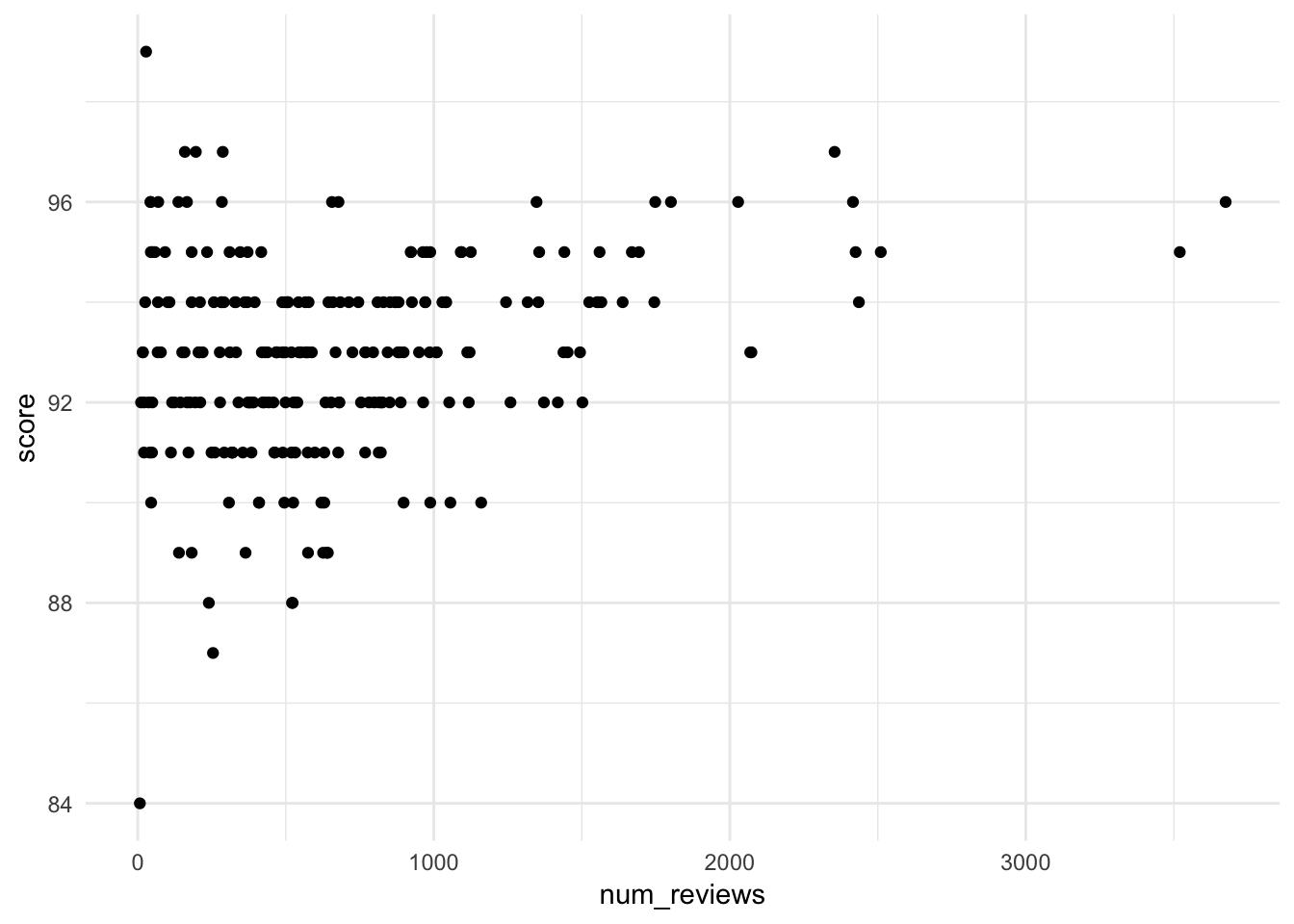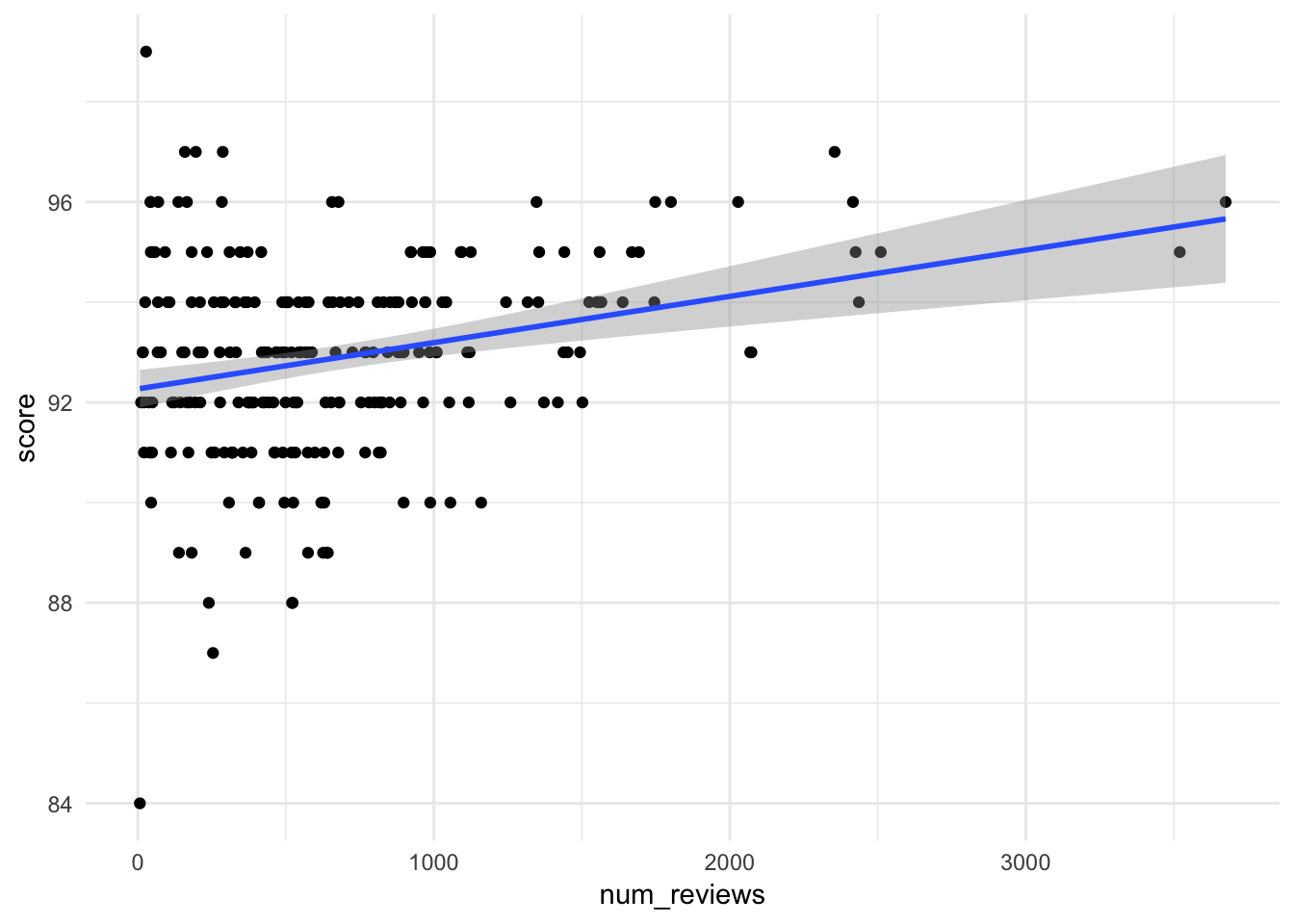You’ll need one new package today, something I wrote called smodels:

``# devtools::install_github("statsmaths/smodels")``

Then, read in the standard libraries.

``````knitr::opts_chunk\$set(echo = TRUE)
library(readr)
library(ggplot2)
library(dplyr)``````
``## Warning: package 'dplyr' was built under R version 3.5.2``
``````library(smodels)
theme_set(theme_minimal())``````

## Tea Reviews

Here, we will take look at a dataset of tea reviews from Adagio Teas:

``tea <- read_csv("https://statsmaths.github.io/stat_data/tea.csv")``
``````## Parsed with column specification:
## cols(
##   name = col_character(),
##   type = col_character(),
##   score = col_double(),
##   price = col_double(),
##   num_reviews = col_double()
## )``````

With the following variables:

• name: the full name of the tea
• type: the type of tea. One of:
• black
• chai
• decaf
• flavors
• green
• herbal
• masters
• matcha
• oolong
• pu_erh
• rooibos
• white
• score: user rated score; from 0 to 100
• price: estimated price of one cup of tea in cents
• num_reviews: total number of online reviews

Draw a scatter plot with num_reviews (x-axis) against score (y-axis):

``````ggplot(tea, aes(num_reviews, score)) +
geom_point()``````Now add a best fit line to the scatter plot:

``````ggplot(tea, aes(num_reviews, score)) +
geom_point() +
geom_bestfit()``````Does the score tend to increase, decrease, or remain the same as the number of reviews increases?

Answer: It increases.

Create a text plot with score (x-axis) against price (y-axis) using the tea name as a label. What is the most expensive tea in the data?

``````ggplot(tea, aes(score, price)) +
geom_text(aes(label = name))``````